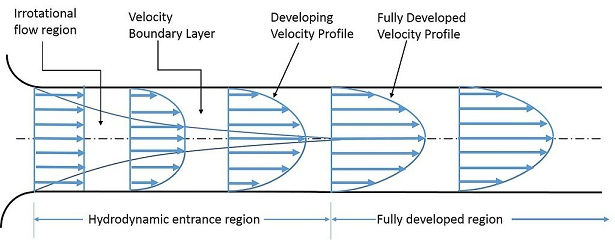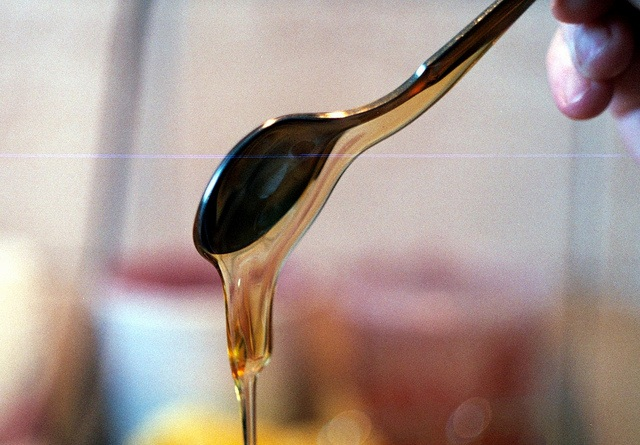# Difference Between Friction and Viscosity

## Main Difference – Friction vs. Viscosity

Friction and viscosity refer to forces that oppose the motion. The main difference between friction and viscosity is that friction is used to refer to forces that resist relative motion, in general, whereas viscosity refers specifically to resistive forces that occur between layers of a fluid when fluids attempt to flow. Therefore, the term “friction” can also refer to resistive forces between solid surfaces, or the resistive forces between solid and fluid surfaces.

## What is Friction

Friction is a term used to refer to forces that resist relative motion in general. Usually, when we use the term “friction”, we refer to dry friction. This is the friction that occurs when two solid surfaces in contact are moving or attempt to move relative to each other. We call it static friction if the surfaces are attempting to move against each other but are not actually moving. When there is relative motion between the surfaces, we call the friction kinetic friction.

The magnitude of friction can be calculated by multiplying the coefficient of friction by the normal contact force between the two surfaces. If the normal contact force is$N$, then the friction$F_r=\mu N$. Once again, if the two surfaces are in motion, the$\mu$ we use is called the coefficient of kinetic friction. When the surfaces are attempting to move against each other but are not in motion, we use the coefficient of kinetic friction.

## What is Viscosity

Viscosity refers to intermolecular forces within a fluid that attempt to resist the motion of the fluid. We can consider fluids to be made of “layers”. When fluid flows, molecules within different layers are moving at different speeds. Consider fluid moving through a circular pipe. At the inner edge of the pipe, there is a boundary layer that flows very slowly because of friction between the walls of the pipe and fluid. Since the boundary layer is flowing very slowly, the intermolecular forces between this layer and the adjacent layer cause the adjacent layer to also flow slowly… and so on. As a result, the layers of fluid closer to the edges of the fluid flow slowly compared to the “inner” layers of fluid. The fastest-flowing layer is the innermost layer of fluid in the pipe.Different layers of fluid flowing through a circular pipe flow at different speeds.

A more viscous fluid flows slowly compared to a fluid with lesser viscosity. The “thicker” a fluid feels, the more viscous it is. For instance, honey is more viscous than water. The viscosity of liquids decreases as temperature rises while, for gases, an increase in temperature is accompanied by an increase in viscosity.Honey is more viscous than water, so it is more difficult to get it to flow.

Superfluids are an interesting type of fluid that shows zero viscosity when cooled to extremely low temperatures. If a superfluid is held inside a container, the fluid can leak through the extremely small pores of the container and “leak out”.

## Difference Between Friction and Viscosity

Friction refers to forces opposing relative motion in general

Viscosity refers specifically to friction occurring between layers of a fluid.

Image Courtesy

“We can see in this image the developing velocity profile of a fluid entering a pipe.” by DEVENDER KUMAR5908 (Own work) [CC BY-SA 3.0], via Wikimedia Commons

“Viscosity Manifest” by Beny Shlevich (Own work) [CC BY 2.0], via flickr